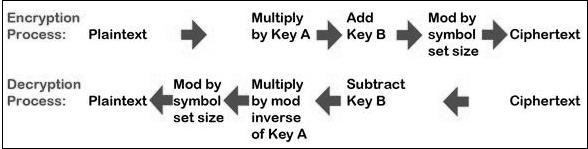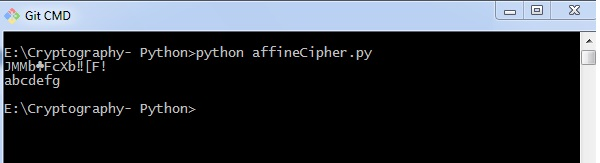# Cryptography with Python – Affine Cipher

Affine Cipher is the combination of Multiplicative Cipher and Caesar Cipher algorithm. The basic implementation of affine cipher is as shown in the image below −In this chapter, we will implement affine cipher by creating its corresponding class that includes two basic functions for encryption and decryption.

## Code

You can use the following code to implement an affine cipher −

```class Affine(object):
DIE = 128
KEY = (7, 3, 55)
def __init__(self):
pass
def encryptChar(self, char):
K1, K2, kI = self.KEY
return chr((K1 * ord(char) + K2) % self.DIE)

def encrypt(self, string):
return "".join(map(self.encryptChar, string))

def decryptChar(self, char):
K1, K2, KI = self.KEY
return chr(KI * (ord(char) - K2) % self.DIE)

def decrypt(self, string):
return "".join(map(self.decryptChar, string))
affine = Affine()
print affine.encrypt('Affine Cipher')
print affine.decrypt('*18?FMT')```

## Output

You can observe the following output when you implement an affine cipher −The output displays the encrypted message for the plain text message Affine Cipher and decrypted message for the message sent as input abcdefg.